# Math in Focus Kindergarten Chapter 14 Answer Key Number Facts

Go through the Math in Focus Grade K Workbook Answer Key Chapter 14 Number Facts to finish your assignments.

## Math in Focus Kindergarten Chapter 14 Answer Key Number Facts

Lesson 1 Number Facts to 10

Count, write, and circle.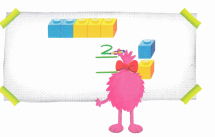Question 1.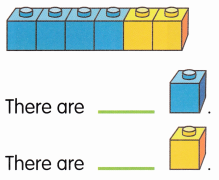How many in all?
2
4
6
Count the blue bottles and yellow bottles.
The total number of blue bottles=4
The total number of yellow bottles=2
The total number of blue and yellow bottles combine=4+2=6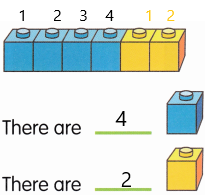Question 2.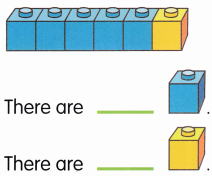How many in all?
3
6
7
Count the blue bottles and yellow bottles.
The total number of blue bottles=5
The total number of yellow bottles=1
The total number of blue and yellow bottles combine=5+1=6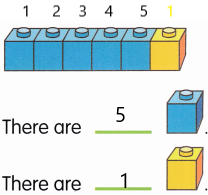Question 3.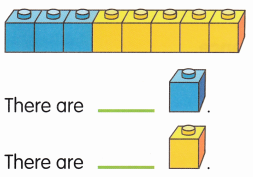How many in all?
8
9
10
Count the blue bottles and yellow bottles.
The total number of blue bottles=3
The total number of yellow bottles=5
The total number of blue and yellow bottles combine=3+5=8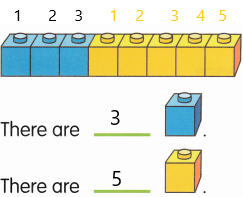Question 4.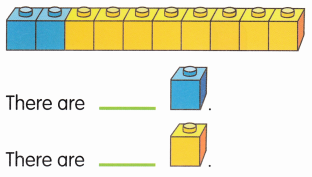How many in all?
7
8
10
Count the blue bottles and yellow bottles.
The total number of blue bottles=2
The total number of yellow bottles=8
The total number of blue and yellow bottles combine=2+8=10

Question 5.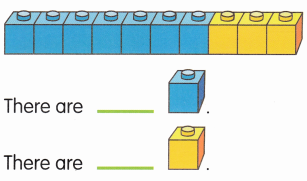How many in all?
10
11
12
Count the blue bottles and yellow bottles.
The total number of blue bottles=7
The total number of yellow bottles=3
The total number of blue and yellow bottles combine=7+3=10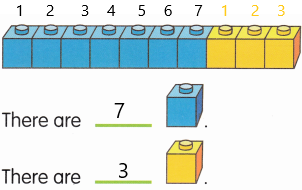Color, count and write. Write the number sentence.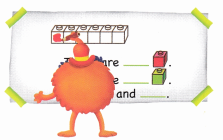Question 1.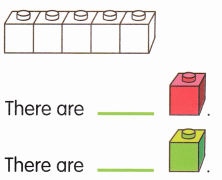5 is _________ and ________.
The sum of two positive integers 3 and 2 is 5
3+2=5
3 and 2 make 5
In the above-given diagram:
The uncoloured boxes are given.
The number of uncoloured boxes=5
We need to colour them red and green.
So we can colour 3 red boxes and 2 green boxes.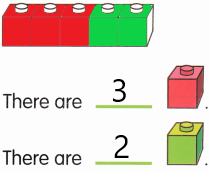Question 2.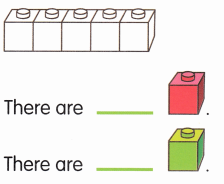5 is _________ and ________.
The sum of two positive integers 4 and 1 is 5
4+1=5
4 and 1 make 5
In the above-given diagram:
The uncoloured boxes are given.
The number of uncoloured boxes=5
We need to colour them red and green.
So we can colour 4 red boxes and 1 green box.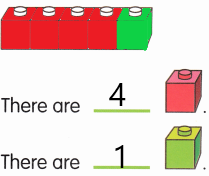Question 3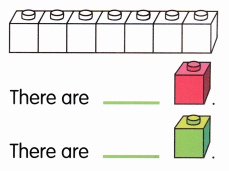7 is _________ and ________.
The sum of two positive integers 3 and 4 is 7
3+4=7
3 and 4 make 7
In the above-given diagram:
The uncoloured boxes are given.
The number of uncoloured boxes=7
We need to colour them red and green.
So we can colour 3 red boxes and 4 green boxes.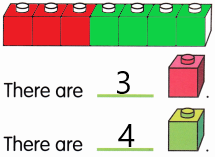Question 4.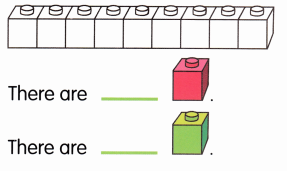9 is _________ and ________.
The sum of two positive integers 4 and 5 is 9
4+5=9
4 and 5 make 9
In the above-given diagram:
The uncoloured boxes are given.
The number of uncoloured boxes=9
We need to colour them red and green.
So we can colour 4 red boxes and 5 green boxes.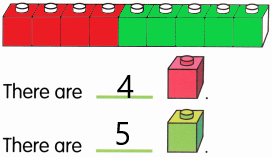Question 5.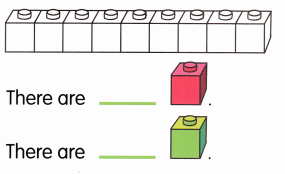9 is _________ and ________.
The sum of two positive integers 8 and 1 is 9
8+1=9
8 and 1 make 9
In the above-given diagram:
The uncoloured boxes are given.
The number of uncoloured boxes=9
We need to colour them red and green.
So we can colour 8 red boxes and 1 green box.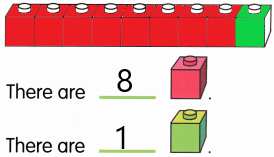Lesson 2 Combining Sets

Count and write.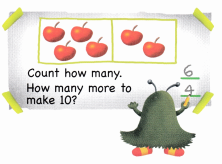Question 1.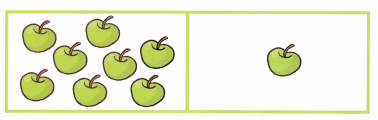Count how many. ____________
How many more to make 10? ___________
The total number of apples=9
We need to find out the number of more apples needed to make 10=X
X=10-9
X=1
Therefore, 1 more apple is needed to make 10.

Question 2.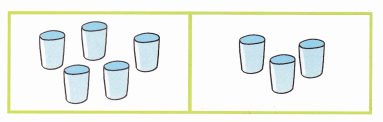Count how many. ____________
How many more to make 10? ___________
The total number of glasses=5+3=8
We need to find out the number of more glasses needed to make 10=X
X=10-8
X=2
Therefore, 2 more glasses are needed to make 10.

Question 3.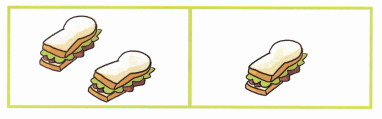Count how many. ____________
How many more to make 10? ___________
The total number of pizzas=2+1=3
We need to find out the number of more pizzas needed to make 10=X
X=10-3
X=7
Therefore, 7 more pizzas are needed to make 10.

Question 4.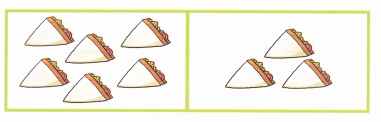Count how many. ____________
How many more to make 10? ___________
The total number of sandwiches=6+3=9
We need to find out the number of more sandwiches needed to make 10=X
X=10-9
X=1
Therefore, 1 more sandwich is needed to make 10.

Question 5.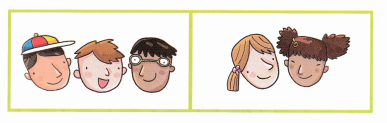Count how many. ____________
How many more to make 10? ___________
The total number of toys=3+2=5
We need to find out the number of more toys needed to make 10=X
X=10-5
X=5
Therefore, 5 more toys are needed to make 10.

Lesson 3 Composing and Decomposing Numbers to 20

Count and write. Write the number sentence.

Question 1.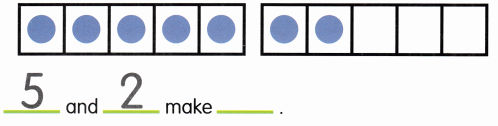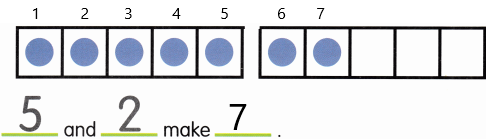5+2=7
The sum of two positive integers 5 and 2 is 7.

Question 2.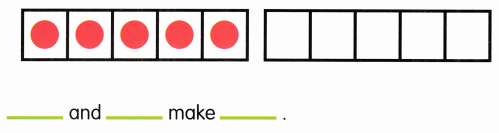_________ and __________ make ___________.
The sum of two positive integers 0 and 5 is 5
0+5=5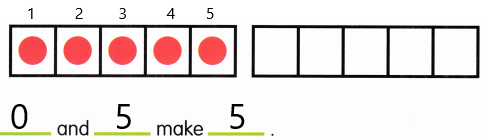Question 3.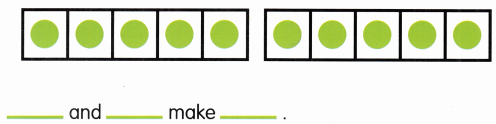_________ and __________ make ___________.
The sum of two positive integers 5 and 5 is 10
5 and 5 make 10.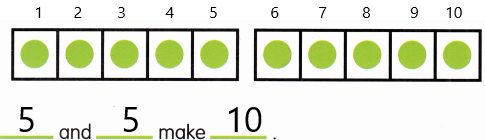Question 4.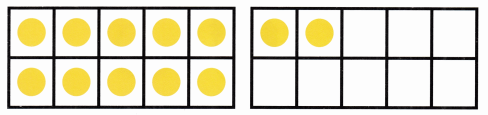_________ and __________ make ___________.
The sum of two positive integers 10 and 2 is 12
10+2=12
10 and 2 make 12.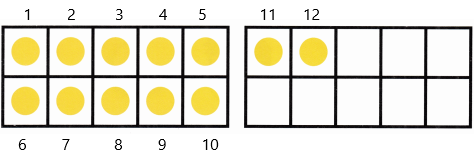Question 5.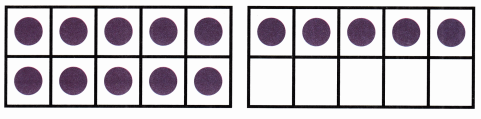_________ and __________ make ___________.
The sum of two positive integers 10 and 5 is 15
10+5=15
10 and 5 make 15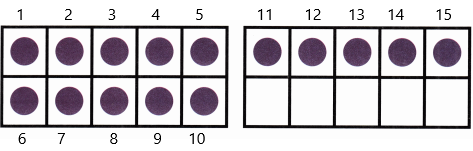Question 6.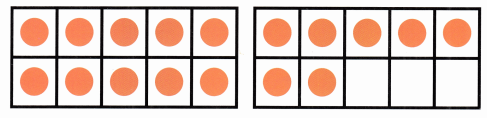_________ and __________ make ___________.
The sum of two positive integers 10 and 7 is 17
10+7=17
10 and 7 make 17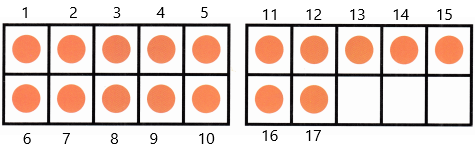Draw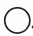. Write the number sentence.

Question 1.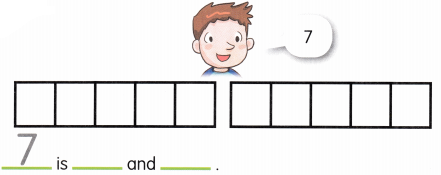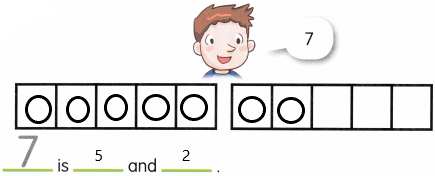5+2=7
So we can write in sentences,
adding 5 and 2 we get 7.

Question 2.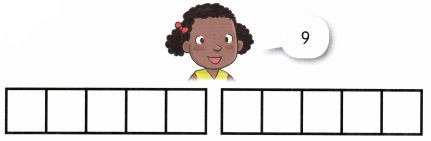__________ is __________ and ___________.
Answer: 9 is 5 and 4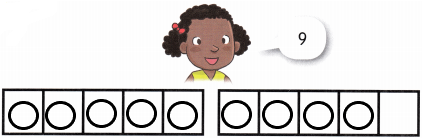5+4=9
And some other ways we can write 9 is:
4+5=9
6+3=9
7+2=9
8+1=9
So we can write in sentences,
by adding above all we get 9.

Question 3.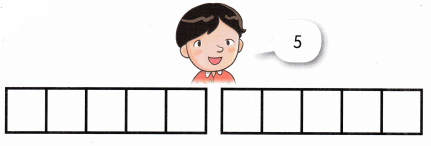__________ is __________ and ___________.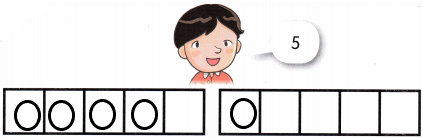5 is 4 and 1
and there are some other ways we can write 5:
2+3=5
3+2=5
4+1=5
So we can write in sentences,
by adding above all we get 5.

Question 4.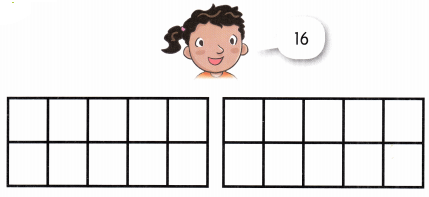__________ is __________ and ___________.
16 is 10 and 6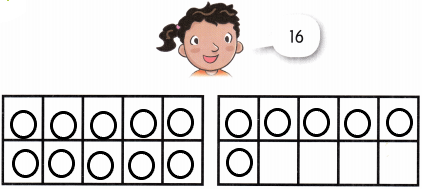and there are some other ways we can write 16:
10+6=16
8+8=16
15+1=16
14+2=16
13+3=16
12+4=16
11+5=16
9+7=16
So we can write in sentences,
by adding above all we get 16.

Question 5.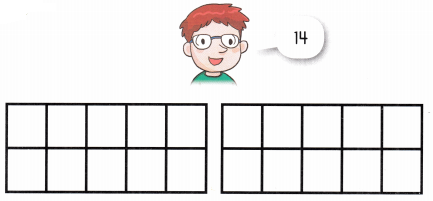__________ is __________ and ___________.
14 is 10 and 4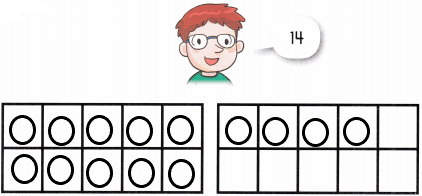and there are some other ways we can write 14:
2+12=14
13+1=14
11+3=14
10+4=14
9+5=14
8+6=14
7+7=14
6+8=14
5+9=14
So we can write in sentences,
by adding above all we get 14.

Lesson 4 Counting On

Count and write.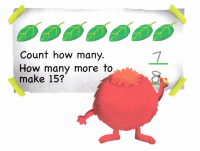Question 1.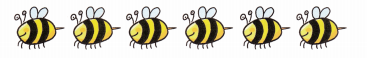Count how many. ____________
How many more to make 15? __________
The number of honey bees = 6
We need to find out the number of more honey bees to make 15=X
X=15-6
X=9
Therefore, 9 more honey bees are needed to make 15.

Question 2.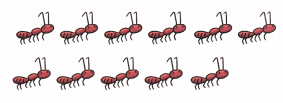Count how many. ____________
How many more to make 15? __________
The number of ants = 11
We need to find out the number of more ants to make 15=X
X=15-11
X=4
Therefore, 4 more ants are needed to make 15.

Question 3.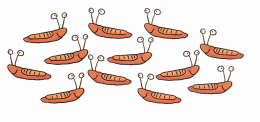Count how many. ____________
How many more to make 15? __________
The number of snails = 12
We need to find out the number of more snails to make 15=X
X=15-12
X=3
Therefore, 3 more snails are needed to make 15.

Question 4.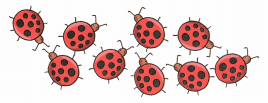Count how many. ____________
How many more to make 15? __________
The number of flies = 9
We need to find out the number of more flies to make 15=X
X=15-9
X=6
Therefore, 6 more flies are needed to make 15.

Question 5.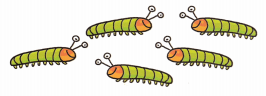Count how many. ____________
How many more to make 15? __________
The number of caterpillars = 5
We need to find out the number of more caterpillars to make 15=X
X=15-5
X=10
Therefore, 10 more caterpillars are needed to make 15.

Count and write.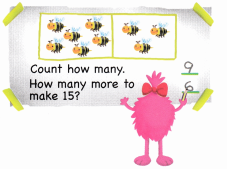Question 1.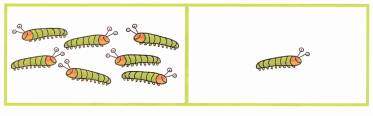Count how many. ____________
How many more to make 15? __________
The number of flies is 8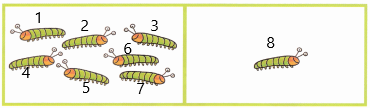The number of flies is needed to make 15 is ‘X’.
X=15-8
X=7
Therefore, 7 more are needed to make 15.

Question 2.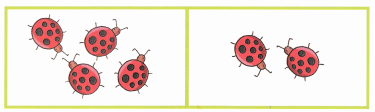Count how many. ____________
How many more to make 15? __________
The number of flies=6
The number of flies is needed to make 15 is ‘X’.
X=15-6
X=9
Therefore, 9 more are needed to make 15.

Question 3.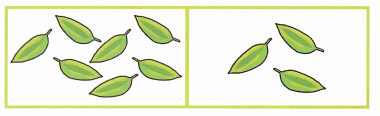Count how many. ____________
How many more to make 15? __________
The number of leaves=10
The number of leaves is needed to make 15 is ‘X’.
X=15-10
X=5
Therefore, 5 more are needed to make 15.

Question 4.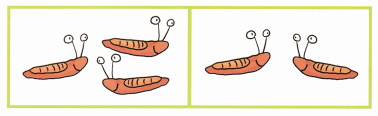Count how many. ____________
How many more to make 15? __________
The number of snails=5
The number of snails is needed to make 15 is ‘X’.
X=15-5
X=10
Therefore, 10 more are needed to make 15.

Question 5.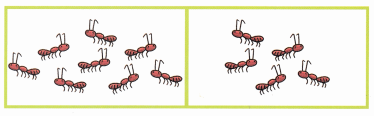Count how many. ____________
How many more to make 15? __________